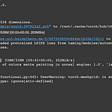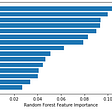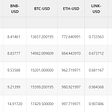# Volatility

Volatility is the standard deviation of probability distribution of log returns. It is the measure of the spread of this particular distribution. Volatility gives a sense of range of values, log returns are likely to fall into.

Volatility can be used for :

• Measuring risks
• Defining position sizes
• Designing alpha factors
• Pricing options

## Historical Volatility

Calculating standard deviation of log returns of past data is known as historical volatility.

## Annualized Volatility

Annualizing volatility means extrapolating standard deviations of log returns calculated at some time frequency to standard deviations of log returns calculated at an annual frequency.

Python implementation of calculating which company has the maximum volatility based on its prices can be found here.

## Conversion between monthly/daily log returns to annual log returns

Below figure represents the conversion formula between monthly/daily log returns to annual log returns. We use 252 while converting a daily log return to yearly log return, because there are 252 trading days in a year!

## Rolling Window

If we want to check how volatility is changing over-time, we use the option of rolling window.

Python implementation of using rolling-window strategy to calculate average can be found here.

## Exponentially Weighted Moving Average

For estimating volatility, we can increase the influence of the more recent past. One common way to do this is to weigh the observation from yesterday, the highest, and then decrease the weights exponentially. This is called exponentially weighted moving average.

The formula for calculating exponentially weighted moving average is as follows :

Here, r_n is the nth daily return and sigma_n is the nth estimate of the volatility. Lambda is a constant between 0 and 1 that defines how quickly weights on older data should decrease.

A high value of lambda (close to 1) will cause older data to matter relatively more in the calculation of sigma_n. A very low value of lambda would mean that the recent data matters more — in this case, the successively daily estimates of sigma_n themselves will be volatile.

# ARCH and GARCH Models

## The ARCH Model

Sometimes, traders try to forecast volatility before it happens. When trying to model volatility, we can use a special form of autoregressive model called ARCH. An ARCH model is used to predict the variance at future time steps.

• ARCH : Autoregressive Conditional Heteroscedastic.
• Autoregressive : Current values are somehow related to recent past values.
• Heteroscedastic : Variable that we are trying to model, may have different magnitudes of variability at different time points. Magnitude of variability is measured using variance. Read more about Heteroskedasticity in my post here.
• In an ARCH model, we must specify a lag parameter (m), to define the number of prior residual errors to include in the model.

ARCH models can be expressed as :

## The GARCH Model

• GARCH : Generalised ARCH
• The GARCH model is an extension of ARCH model. It includes lag residual (m) terms together with lag variance terms (n). It incorporates moving average component together with the autoregressive component.
• A typical GARCH model can be expressed as the figure below. An example of GARCH model of the first order could be expressed as GARCH(1,1). A GARCH(0, m) model is equivalent to ARCH(m) model.

--

--

--

## More from The Startup

Get smarter at building your thing. Follow to join The Startup’s +8 million monthly readers & +756K followers.

## Making an AI to create beautiful artistic animations.(Part-2 Intermediate Method using VQGAN+CLIP)## Replicating human mind. Where are we lacking?## How Intelligent Virtual Assistants Help Brands — Haptik Blog## 7 Remarkable IVR Trends For the Year 2021 And Beyond## Entale AI gets an upgrade## My first few weeks into the worlds most powerful AI language generating model — OpenAI’s GPT-3## Ivy is a 24-hour virtual concierge service that smooths the stay of guests at hotels (Raj Singh)## An AI breakthrough that may mean curtains for poets, journalists…and me## Purva Singh

Hi! I am a tech enthusiast currently working on leveraging language technologies to solve financial use-cases! View my work here: https://purvasingh96.github.io

## Introduction to Market Structure in Trading## Momentum strategy — ML in the Crypto environment## Standard market methods for optimizing the cryptocurrency portfolio.## How GPT-3 changes everything